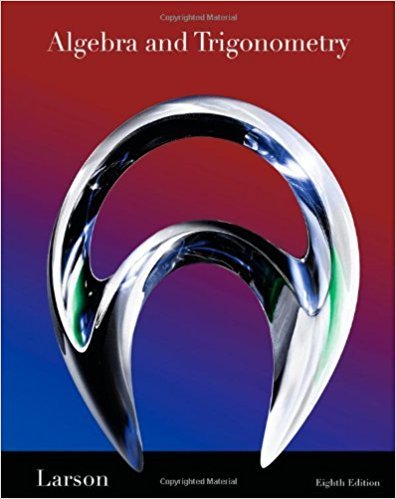×
Get Full Access to Algebra And Trigonometry - 8 Edition - Chapter 9.5 - Problem 3
Get Full Access to Algebra And Trigonometry - 8 Edition - Chapter 9.5 - Problem 3

×

# The graph of a ________ inequality is a half-plane lyingISBN: 9781439048474 185

## Solution for problem 3 Chapter 9.5

Algebra and Trigonometry | 8th Edition

• Textbook Solutions
• 2901 Step-by-step solutions solved by professors and subject experts
• Get 24/7 help from StudySoup virtual teaching assistantsAlgebra and Trigonometry | 8th Edition

4 5 1 252 Reviews
26
3
Problem 3

The graph of a ________ inequality is a half-plane lying on one side of the line

Step-by-Step Solution:
Step 1 of 3

19/01/2017 Chapter 1: Functions and their Graphs 1.2 Graphs of a function - Linear equation: y = 2x+1 X Y 0 1 -4 -7 100 201 - Quadratic equation: y = x - 2 X Y 2 2 0 -2 -2 2 -3 7 3 7 rd 3 - 3 degree equation: y = x X Y

Step 2 of 3

Step 3 of 3

##### ISBN: 9781439048474

Unlock Textbook Solution